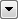# StatisticsCalculator

Calculates statistics based on a designated attribute or set of attributes of the incoming features and adds the results as attributes.

### Typical Uses

• Inspecting and analyzing features
• Calculating statistics for use in further operations

## How does it work?

The StatisticsCalculator receives features and calculates selected statistics on them per attribute. Statistics can be calculated on specific groups of input features as specified by the Group By attribute option. The results are output on each feature out of the Complete port. A summary of the results is output out of the Summary port.

Available statistics include:

• Minimum
• Maximum
• Total Count
• Sum
• Mean
• Median
• Numeric Count
• Value Count
• Range
• Standard Deviation (Sample or Population)
• Mode
• Histogram

The following statistics may also be calculated cumulatively on attributes using advanced settings: min, max, range, mean, stdev, sum, total count, numeric count, and value count. When calculating cumulative statistics, the current results thus far are output on each feature out of the Cumulative port.

Statistics are stored as attributes, named <attribute>.<statisticname>, where <statisticname> will be one of the following: min, max, range, mean, stdev, stdev_p, sum, median, mode, total_count, numeric_count, value_count, histogram.

Histogram statistics are stored as list attributes that pair the attribute value and count for each unique value of the attribute and are named <attribute>.histogram{#}.value and <attribute>.histogram{#}.count where # is a zero-based index of the unique attribute values.

### Input Data Handling

Invalid, <null>, and <missing> data are considered invalid and will be skipped when calculating statistics other than total count. If no valid values are processed when calculating a statistic the result will be <missing>. Trying to calculate standard deviation on only one value will also result in <missing>. Total count, numeric count, and value count will never be <missing>. NaN values are explicitly ignored when calculating Min, Max, Range, and Numeric Count.

For example, a calculated sum on all <missing> values will be <missing> rather than a potentially misleading and less informative 0. However, if only some values are <missing> or invalid the resulting sum will be the same as if those values were 0.

## Usage Notes

• The StatisticsCalculator transformer has default suffixes and always prepends. Upgraded transformers will retain prepend settings when output attribute names were not prepended before upgrade as well as suffix names to maintain backwards compatibility and avoid disrupting existing workspaces.

## Editing Transformer Parameters

Using a set of menu options, transformer parameters can be assigned by referencing other elements in the workspace. More advanced functions, such as an advanced editor and an arithmetic editor, are also available in some transformers. To access a menu of these options, clickbeside the applicable parameter. For more information, see Transformer Parameter Menu Options.

### Defining Values

There are several ways to define a value for use in a Transformer. The simplest is to simply type in a value or string, which can include functions of various types such as attribute references, math and string functions, and workspace parameters. There are a number of tools and shortcuts that can assist in constructing values, generally available from the drop-down context menu adjacent to the value field.

### Dialog Options - Tables

Transformers with table-style parameters have additional tools for populating and manipulating values.

## FME Community

The FME Community is the place for demos, how-tos, articles, FAQs, and more. Get answers to your questions, learn from other users, and suggest, vote, and comment on new features.